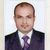QlikView App Dev

Discussion Board for collaboration related to QlikView App Development.

Announcements
QlikWorld 2022, LIVE in Denver CO., May 16-19, 2022. REGISTER NOW TO RECEIVE EARLY BIRD PRICING
cancel
Showing results for
Did you mean:Not applicable

LET and SET

Hi

What is the use of LET and SET? What is the difference between them?

Thanks

Attitude

7 RepliesEmployee

Hi,

As you can see in the Reference Manual, they are used to assign values to variables in the load script. The main difference is that while SET assigns the value to the variable without evaluation, as a string, the LET first evaluates the expression at the right part of the "=" sign, then stores the value.

Two simple examples:

SET vLiteral = 1 + 1; // This stores the string "1 + 1";

LET vSum = 1 + 1; // This stores 2

Check for further reference the Manual and this thread on how they work and when and how to expand and this other one among many others.

Hope that helps.

Miguel Angel Baeyens

BI Consultant

Comex Grupo IbéricaMVP

Hi,

The Set command assigns the variable the text to the right of the equal sign, whereas the Let command evaluates the expression

set variablename = string

let variable = expression

Rgds

AnandNot applicable
Author

Hi,

Let and Set commands are used to define Script Variables. Any text or numeric value can be assigned to the script variables.

Examples:

1.

Set V = \$ ; ( Set- assigns the variable(V) the text(String) to the right of the equal sign)

Let vStart = num(makedate(2011)); (Let- evaluates the expression)

2.

Set MUL = ’\$1*\$2’;

Set X = \$(MUL(3,7));   // returns '3*7' in X

Let X = \$(MUL(3,7));   // returns 21 in X

Regards

ApurvaMVP

Hi,

See the example like

1.

Let T=now( );

It will give you exact time because the value of now( ) return value assign to Let T variable and it retun time value.

2.

Set P=now( );

But SET will give and return the now( )

means what ever you write for code it accept it as string

Rgds

AnandMaster

Set x=3+4;

Let y=3+4

z=\$(y)+1;

\$(x) will be evaluated as ' 3+4 '

\$(y) will be evaluated as ' 7 '

\$(z) will be evaluated as ' 8 'Partner

Hi

It is very simple:

using SET you are adding string value to a variable
SET Variable1='4+5';
variable value is '4+5'

using LET you are adding an expression result to a variable
LET Variable1=4+5;
variable value is 9Not applicable
Author

Hi,

In Qlikview  there are two variable SET and LET.The difference between these two is that the SET statement assigns literal string to SET variable,where LET Statement first evaluate the string before assigning it

e.g.

Statement                                     Value of variable

Set aVariable= 2+3;                          2+3;

Let bVariable = 2+3;                            5;Community Browser